Note: The other languages of the website are Google-translated. Back to English

## Excel COUNTIF function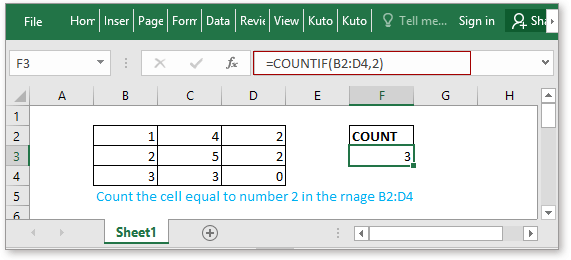### Description

The COUNTIF function is a statistical function in Excel which is used to count the number of cells that meet a criterion. It supports logical operators (<>, =, >, and <), and the wildcards (? and *) for partial matching.

### Syntax and arguments

Formula syntax

 COUNTIF(range,criteria)

Arguments

 Range: Required, the range of cells that you want to count. Criteria: Required, the criterion that you count cells based to.

Return Value

The COUNTIF function returns the number of cells that meeting the specified criteria.

### Basic Usage

Example1 Count cells equal to

Supposing you want to count the number of cells that equal to 2, you can use the formula as below:

 =COUNTIF(B2:D4,2)

Or

 =COUNTIF(B2:D4,G3)

Press Enter key to return the number of cells that equal to 2.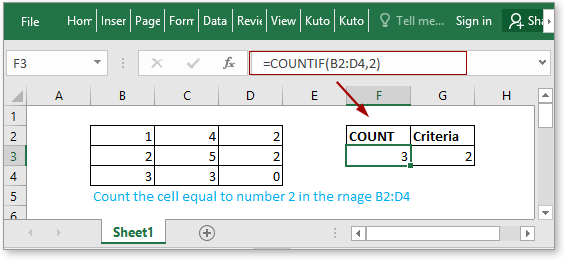For more usages about counting cells exactly equal to and partial equal to, please click here to see the formulas and examples.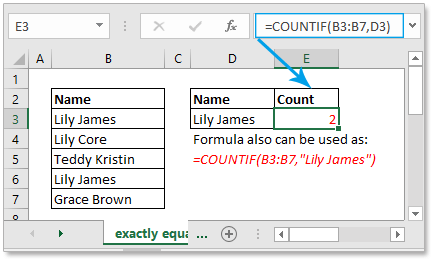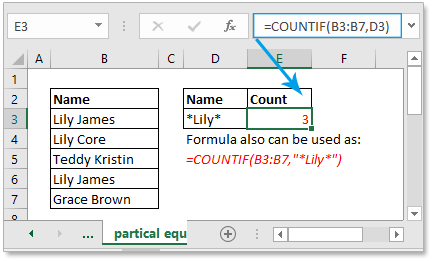Example2 Count the cells greater than or less than

If you want to count cells that are greater than or less than a given number, you also can use the COUNTIF function.

Supposing there is a range (B2:D5) of numbers, you want to count the number of cells that are greater than 65, please using below formula:

 =COUNTIF(B2:D5,">65")

Or

 =COUNTIF(B2:D5,">65")

Press Enter key to get the result.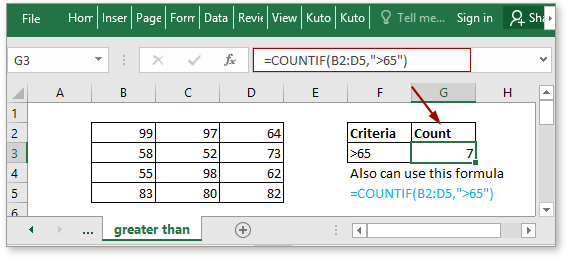If you want to count cells that are less than a number, for example, count cells less than 50, just use the formula like this =COUNTIF(B2:D5,"<50 "), click here for more details about counting cells greater than or less than a given value.

Example1 Count cells not equal to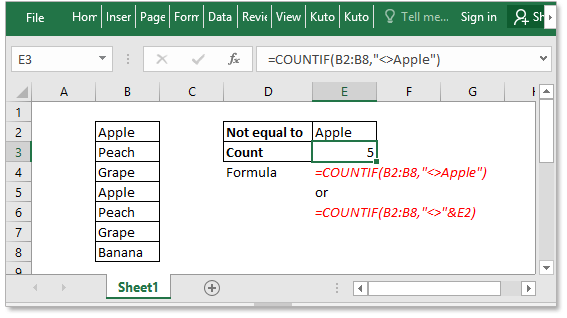Example2 Count cells equal to x or y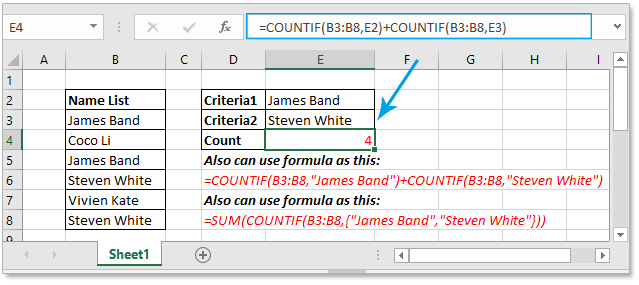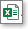Click to download COUNTIF function sample file

#### Relative Functions:

• Excel COUNTA Function
The COUNTA function returns the number of cells that contain number, text, error value or the empty text “”, and excluding the real empty cells.

• Excel COUNTBLANK Function
The COUNTBLANK function returns the number of empty cells. The COUNTBLANK function is a built-in statistical function, which can be used as a worksheet function (WS) in Excel.

• Excel COUNT Function
The COUNT function is used to count the number of cells that contain numbers, or count the numbers in a list of arguments. Take an instance, COUNT(1,2,3,4,11,2,1,0) returns 8.

• Excel COUNTIFS Function
The COUNTIFS function returns the number of cells that meet one single criterion or multiple criteria. The COUNNTIFS function is one of the built-in statistical function, and it can be used as a worksheet function (WS) in Excel.

#### Relative Articles:

• Count cells equal to
If you want to count cells exactly match a given criterion, you can choose the COUNTIF function or the SUMPRODUCT (combine with the EXACT function) function to count cells exactly equal to without case sensitive or with case sensitive.

• Count cells not equal to
To count the number of cells that contain values that not equal to a specified value, such as below screenshot shown. In Excel, you can use the COUNTIF function to quickly handle this job.

• Count cells equal to x or y
If you want to count cells as long as that meet at least one of given conditions (OR logic) in Excel, i.e. count cells equal to either x or y, you can use several COUNTIF formulas or combine the COUNTIF formula and SUM formula to handle this job.

### The Best Office Productivity Tools

#### Kutools for Excel - Helps You To Stand Out From Crowd

Would you like to complete your daily work quickly and perfectly? Kutools for Excel brings 300 powerful advanced features (Combine workbooks, sum by color, split cell contents, convert date, and so on...) and save 80% time for you.

• Designed for 1500 work scenarios, helps you solve 80% Excel problems.
• Reduce thousands of keyboard and mouse clicks every day, relieve your tired eyes and hands.
• Become an Excel expert in 3 minutes. No longer need to remember any painful formulas and VBA codes.
• 30-day unlimited free trial. 60-day money back guarantee. Free upgrade and support for 2 years.#### Office Tab - Enable Tabbed Reading and Editing in Microsoft Office (include Excel)

• One second to switch between dozens of open documents!
• Reduce hundreds of mouse clicks for you every day, say goodbye to mouse hand.
• Increases your productivity by 50% when viewing and editing multiple documents.
• Brings Efficient Tabs to Office (include Excel), Just Like Chrome, Firefox, And New Internet Explorer.